Home Practice
For learners and parents For teachers and schools
Textbooks
Full catalogue
Pricing SupportLog in

We think you are located in United States. Is this correct?

# 3.2 Molecular shape

## 3.2 Molecular shape (ESBM9)

Molecular shape (the shape that a single molecule has) is important in determining how the molecule interacts and reacts with other molecules. Molecular shape also influences the boiling point and melting point of molecules. If all molecules were linear then life as we know it would not exist. Many of the properties of molecules come from the particular shape that a molecule has. For example if the water molecule was linear, it would be non-polar and so would not have all the special properties it has.

### Valence shell electron pair repulsion (VSEPR) theory (ESBMB)

The shape of a covalent molecule can be predicted using the Valence Shell Electron Pair Repulsion (VSEPR) theory. Very simply, VSEPR theory says that the valence electron pairs in a molecule will arrange themselves around the central atom(s) of the molecule so that the repulsion between their negative charges is as small as possible.

In other words, the valence electron pairs arrange themselves so that they are as far apart as they can be.

Valence Shell Electron Pair Repulsion Theory

Valence shell electron pair repulsion (VSEPR) theory is a model in chemistry, which is used to predict the shape of individual molecules. VSEPR is based upon minimising the extent of the electron-pair repulsion around the central atom being considered.

VSEPR theory is based on the idea that the geometry (shape) of a molecule is mostly determined by repulsion among the pairs of electrons around a central atom. The pairs of electrons may be bonding or non-bonding (also called lone pairs). Only valence electrons of the central atom influence the molecular shape in a meaningful way.

### Determining molecular shape (ESBMC)

To predict the shape of a covalent molecule, follow these steps:

1. Draw the molecule using a Lewis diagram. Make sure that you draw all the valence electrons around the molecule's central atom.
2. Count the number of electron pairs around the central atom.
3. Determine the basic geometry of the molecule using the table below. For example, a molecule with two electron pairs (and no lone pairs) around the central atom has a linear shape, and one with four electron pairs (and no lone pairs) around the central atom would have a tetrahedral shape.

The central atom is the atom around which the other atoms are arranged. So in a molecule of water, the central atom is oxygen. In a molecule of ammonia, the central atom is nitrogen.

The table below gives the common molecular shapes. In this table we use A to represent the central atom, X to represent the terminal atoms (i.e. the atoms around the central atom) and E to represent any lone pairs.

 Number of bonding electron pairs Number of lone pairs Geometry General formula $$\text{1}$$ or $$\text{2}$$ $$\text{0}$$ linear $$\text{AX}$$ or $$\text{AX}_{2}$$ $$\text{2}$$ $$\text{2}$$ bent or angular $$\text{AX}_{2}\text{E}_{2}$$ $$\text{3}$$ $$\text{0}$$ trigonal planar $$\text{AX}_{3}$$ $$\text{3}$$ $$\text{1}$$ trigonal pyramidal $$\text{AX}_{3}\text{E}$$ $$\text{4}$$ $$\text{0}$$ tetrahedral $$\text{AX}_{4}$$ $$\text{5}$$ $$\text{0}$$ trigonal bipyramidal $$\text{AX}_{5}$$ $$\text{6}$$ $$\text{0}$$ octahedral $$\text{AX}_{6}$$
Table 3.1: The effect of electron pairs in determining the shape of molecules. Note that in the general example $$\text{A}$$ is the central atom and $$\text{X}$$ represents the terminal atoms.

In Figure 3.7 the green balls represent the lone pairs (E), the white balls (X) are the terminal atoms and the red balls (A) are the center atoms.

Of these shapes, the ones with no lone pairs are called the ideal shapes. The five ideal shapes are: linear, trigonal planar, tetrahedral, trigonal bipyramidal and octahedral.

One important point to note about molecular shape is that all diatomic (compounds with two atoms) compounds are linear. So $$\text{H}_{2}$$, $$\text{HCl}$$ and $$\text{Cl}_{2}$$ are all linear.

## Worked example 6: Molecular shape

Determine the shape of a molecule of $$\text{BeCl}_{2}$$

### Draw the molecule using a Lewis diagram

The central atom is beryllium.

### Count the number of electron pairs around the central atom

There are two electron pairs.

### Determine the basic geometry of the molecule

There are two electron pairs and no lone pairs around the central atom. $$\text{BeCl}_{2}$$ has the general formula: $$\text{AX}_{2}$$. Using this information and Table 3.1 we find that the molecular shape is linear.

The molecular shape of $$\text{BeCl}_{2}$$ is linear.

## Worked example 7: Molecular shape

Determine the shape of a molecule of $$\text{BF}_{3}$$

### Draw the molecule using a Lewis diagram

The central atom is boron.

### Count the number of electron pairs around the central atom

There are three electron pairs.

### Determine the basic geometry of the molecule

There are three electron pairs and no lone pairs around the central atom. The molecule has the general formula $$\text{AX}_{3}$$. Using this information and Table 3.1 we find that the molecular shape is trigonal planar.

The molecular shape of $$\text{BF}_{3}$$ is trigonal planar.

## Worked example 8: Molecular shape

Determine the shape of a molecule of $$\text{NH}_{3}$$

### Count the number of electron pairs around the central atom

There are four electron pairs.

### Determine the basic geometry of the molecule

There are three bonding electron pairs and one lone pair. The molecule has the general formula $$\text{AX}_{3}\text{E}$$. Using this information and Table 3.1 we find that the molecular shape is trigonal pyramidal.

The molecular shape of $$\text{NH}_{3}$$ is trigonal pyramidal.

We can also work out the shape of a molecule with double or triple bonds. To do this, we count the double or triple bond as one pair of electrons.

temp text

## Building molecular models

In groups, you are going to build a number of molecules using jellytots to represent the atoms in the molecule, and toothpicks to represent the bonds between the atoms. In other words, the toothpicks will hold the atoms (jellytots) in the molecule together. Try to use different coloured jellytots to represent different elements.

You will need jellytots, toothpicks, labels or pieces of paper.

On each piece of paper, write the words: “lone pair”.

You will build models of the following molecules:

$$\text{HCl}$$, $$\text{CH}_{4}$$, $$\text{H}_{2}\text{O}$$, $$\text{BF}_{3}$$, $$\text{PCl}_{5}$$, $$\text{SF}_{6}$$ and $$\text{NH}_{3}$$.

For each molecule, you need to:

• Determine the molecular geometry of the molecule

• Build your model so that the atoms are as far apart from each other as possible (remember that the electrons around the central atom will try to avoid the repulsions between them).

• Decide whether this shape is accurate for that molecule or whether there are any lone pairs that may influence it. If there are lone pairs then add a toothpick to the central jellytot. Stick a label (i.e. the piece of paper with “lone pair” on it) onto this toothpick.

• Adjust the position of the atoms so that the bonding pairs are further away from the lone pairs.

• How has the shape of the molecule changed?

• Draw a simple diagram to show the shape of the molecule. It doesn't matter if it is not $$\text{100}\%$$ accurate. This exercise is only to help you to visualise the 3-dimensional shapes of molecules. (See Figure 3.7 to help you).

Do the models help you to have a clearer picture of what the molecules look like? Try to build some more models for other molecules you can think of.

## Molecular shape

Textbook Exercise 3.6

Determine the shape of the following molecules.

$$\text{BeCl}_{2}$$

The central atom is beryllium (draw the molecules Lewis structure to see this).

There are two electron pairs around beryllium and no lone pairs.

There are two bonding electron pairs and no lone pairs. The molecule has the general formula $$\text{AX}_{2}$$. Using this information and Table 3.1 we find that the molecular shape is linear.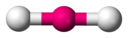$$\text{F}_{2}$$

The molecular shape is linear. (This is a diatomic molecule)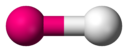$$\text{PCl}_{5}$$

The central atom is phosphorous.

There are five electron pairs around phosphorous and no lone pairs.

The molecule has the general formula $$\text{AX}_{5}$$. Using this information and Table 3.1 we find that the molecular shape is trigonal bipyramidal.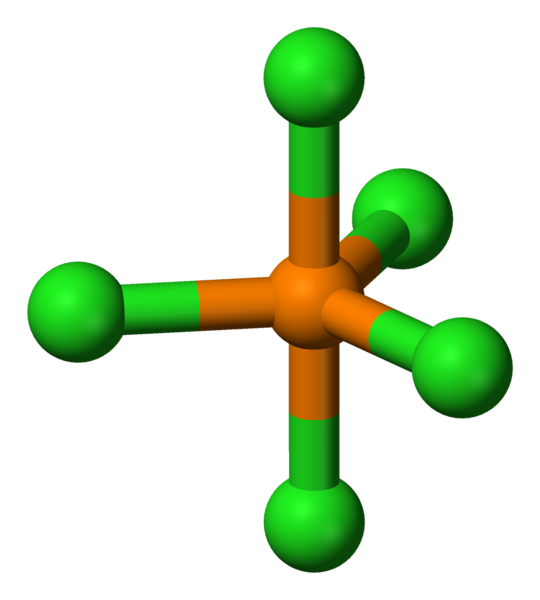$$\text{SF}_{6}$$

The central atom is sulfur.

There are six electron pairs around phosphorous and no lone pairs.

The molecule has the general formula $$\text{AX}_{6}$$. Using this information and Table 3.1 we find that the molecular shape is octahedral.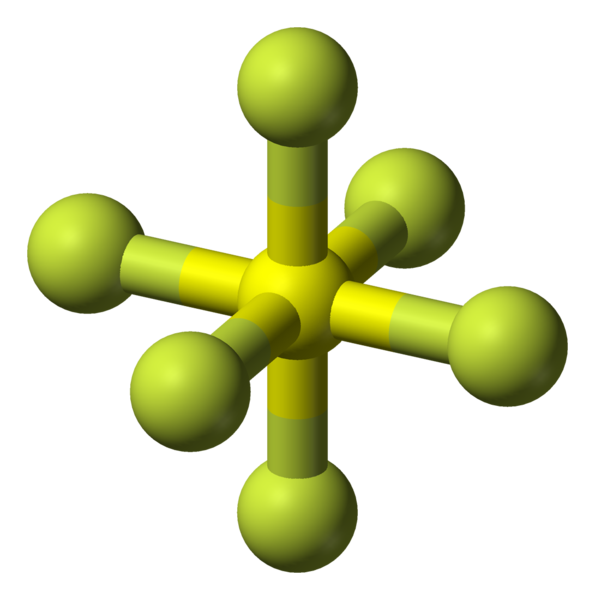$$\text{CO}_{2}$$

The central atom is carbon. (Draw the molecules Lewis diagram to see this.)

There are four electron pairs around carbon. These form two double bonds. There are no lone pairs around carbon.

The molecule has the general formula $$\text{AX}_{2}$$. Using this information and Table 3.1 we find that the molecular shape is linear.$$\text{CH}_{4}$$

The central atom is carbon. (Draw the molecules Lewis diagram to see this).

There are four electron pairs around carbon and no lone pairs.

The molecule has the general formula $$\text{AX}_{4}$$. Using this information and Table 3.1 we find that the molecular shape is tetrahedral.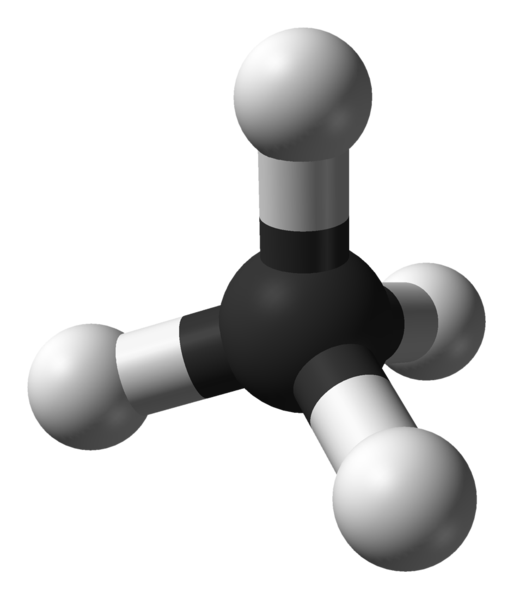$$\text{H}_{2}\text{O}$$

The central atom is oxygen (you can see this by drawing the molecules Lewis diagram).

There are two electron pairs around oxygen and two lone pairs.

The molecule has the general formula $$\text{AX}_{2}\text{E}_{2}$$. Using this information and Table 3.1 we find that the molecular shape is bent or angular.$$\text{COH}_{2}$$

The central atom is carbon.

There are four electron pairs around carbon, two of which form a double bond to the oxygen atom. There are no lone pairs.

The molecule has the general formula $$\text{AX}_{3}$$. Using this information and Table 3.1 we find that the molecular shape is trigonal planar.